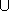## PolygonConvexDecomposition_2

A model of the PolygonConvexDecomposition_2 concept is capable of decomposing an input polygon P into a set of convex sub-polygons P1, ..., Pk, such thati=1kPk = P.

### Types

 PolygonConvexDecomposition_2::Kernel the geometric kernel type. PolygonConvexDecomposition_2::Point_2 the point type, used to represent polygon vertices. PolygonConvexDecomposition_2::Polygon_2 the polygon type.

### Creation

 PolygonConvexDecomposition_2 decomp; default constructor.

### Operations

 template OutputIterator decomp ( Polygon_2 P , OutputIterator oi ) decomposes the input polygon P into convex sub-polygons, and writes them to the output iterator oi. The value-type of the output iterator must be Polygon_2. The function returns a past-the-end iterator for the convex sub-polygons.

### Has Models

Small_side_angle_bisector_decomposition_2<Kernel,Container>
Optimal_convex_decomposition_2<Kernel,Container>
Hertel_Mehlhorn_convex_decomposition_2<Kernel,Container>
Greene_convex_decomposition_2<Kernel,Container>